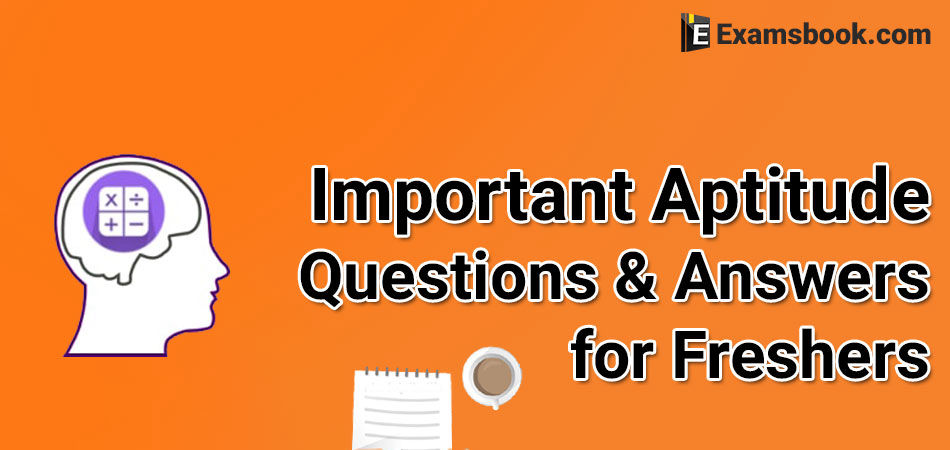• Save

# General Aptitude Questions and Answers for Freshers for Competitive ExamsStudents who are appearing for the first time in the competitive exam or are preparing for the competitive exams, important general aptitude questions and answers for freshers are being given in this blog. These general aptitude questions are important to the every examination.

Most of these questions have been asked in the previous competitive exams. So you should constantly practice these aptitude questions and strengthen your preparation to score high in your competitive exams.

Here you can look selective general aptitude questions for better preparation as well as General aptitude test questions by one click.

## Important Aptitude Questions and Answers for Freshers:

Q.1. A and B together can finish a work in 30 days. They worked for it for 20 days and then B left the work. The remaining work was done by A alone in 20 days more. In how many days can A alone finish the work?

(A) 48 days
(B) 50 days
(C) 54 days
(D) 60 days

Ans .   D

Q.2. A merchant changed his trade discount from 25% to 15%. This would increase selling price by

(A) 3⅓%
(B) 6⅙%
(C) 13⅓%
(D) 16⅓%

Ans .   C

Q.3. If percentage of profit made, when an article is sold for Rs.78, is twice as when it is sold for Rs.69, the cost price of the article is

(A) Rs. 49
(B) Rs. 51
(C) Rs. 57
(D) Rs. 60

Ans .   D

Q.4.Gautam travels 160 kms at 32 kmph and returns at 40 kmph. Then average speed is

(A) 72 kmph
(B) 71.11 kmph
(C) 36 kmph
(D) 35.55 kmph

Ans .   D

Q.5.If a+b+c = 6 and ab+bc+ca = 1, then the value of bc(b+c) + ca(c+a) +ab(a+b) +3abc is

(A) 33
(B) 66
(C) 55
(D) 23

Ans .   B

Q.6.In ΔABC, ∠A = 90°, AD ┴ BC and AD = BD = 2 cm. The length of CD is

(A) 3 cm
(B) 3.5 cm
(C) 3.2 cm
(D) 2 cm

Ans .   D

Q.7.(251 + 252+253+254+255) is divisible by

(A) 23
(B) 58
(C) 124
(D) 127

Ans .   C

$$Q.8. \ If \left(a+{1\over a} \right)^2=3, then \ the \ value\ of \ a^6-{1\over a^6} will \ be$$

(A) 1
(B) 3
(C) 0
(D) 2

Ans .   C

Q.9.The perimeter of two similar triangles ABC and PQR are 36 cms and 24 cms respectively. If PQ = 10 cm then the length of AB is

(A) 18 cm
(B) 12 cm
(C) 15 cm
(D) 30 cm

Ans .   C

Q.10. ABC is triangle If Sin (A+B/ 2) =√3/2, then the value of Sin C/2 is

(A) 1/√2

(B) 0

(C) 1/2

(D) √3/2

Ans .   D

You can ask me freely anything in the comment section related to general aptitude questions, if you have any doubt or problem. Go to the next page for more practice of these questios with answers.